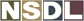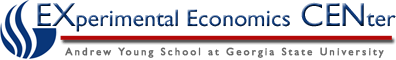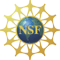Students / Subjects# Representation of Preferences by a Utility Function

A consumer's preferences can be represented by a utility function if they satisfy properties P.1 through P.4, and one additional property called continuity. Continuity is probably the least intuitive property of preferences, yet it is not implausible.

### P.5 The "Continuity" Property

Preferences are continuous if the set of all choices that are at least as good as a choice x' and the set of all choices that are no better than x' are both closed sets. In the notation of sets, this is written as {x : x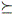x'} and {x : x'x} are both closed.

One definition of a closed set is that any sequence of points in the set that converges, converges to a point of the set. In this context, that means that for a sequence of points {xn} with n = 1, 2, 3, ..., if xxn for every xn and if xn converges to some consumption point x', then xx'.

Figure 6 shows an example of this. In the figure, if xxn for every n, and if xn converges to x', then continuity implies that xx'.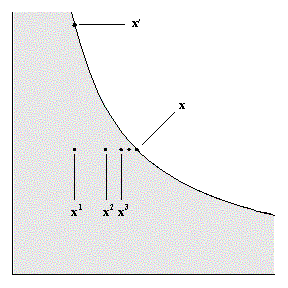Figure 6: Sequence of points xn that converge to x'.

### Representation Theorem

If a consumer has a preference relation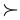that is complete, reflexive, transitive, strongly monotonic, and continuous, then these preferences can be represented by a continuous utility function u(x) such that u(x) > u(x') if and only if xx'.

Proof : Let e = (1, 1, ..., 1). For each x, define u(x) by u(x) e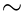x. Then u(x) is a utility function for the preferencesif
1. such a function u(x) exists,
2. the function u(x) is unique, and
3. u(x) > u(x') if and only if xx'.

Let B = {a: a ex}. If x = (x1, x2), let y = (max{x1, x2}, max{x1, x2}). Then strong monotonicity implies that yx, so B is not empty. Let W = {a: xa e}. Then 0 is an element of W, so W is not empty. By completeness, B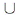W = {a : a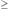0}. Both B and W are closed sets, from property P.5 (continuity), so B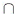W is not empty. Therefore, there is some a such that a ex. By strong monotonicity, if a' > a, then a' ex, and if a' < a, then xa' e, so a is unique. Let u(x) = a. So u(x) exists and it is unique.

Next, we want to show that u(x) represents the preferences. Suppose that x and y are two consumption levels and u(x) = ax where a x ex. Let u(y) = a y where a y ey. If a x > a y then by monotonicty a x ea y e. By transitivity, xa x ea y ey. Finally, if xy, then a x ea y e so that a x > a y.

Next